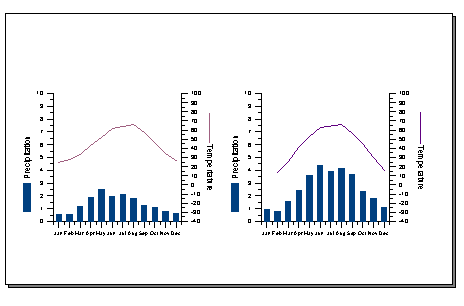# Creating Multiple Graphs on a Page

There are several ways to create more than one graph on a page. The easiest method is to use the mouse, although you can edit the axis properties when creating multiple graphs on a page.

To create multiple graphs on a page:

1. Create the first graph.
2. Select the entire graph.
3. Drag the graph to a new position using the mouse.
4. Create the second graph.
5. Select the entire second graph.
6. Drag the graph to a new position using the mouse.

The graph can also be moved and sized using the axis properties.To create graphs similar to the one shown above on Legal size paper:

1. Create the first graph.
2. Edit the axis properties. In the left graph, the length and starting positions were set as follows:
• Y Axis 1 (left): Length 4.00 inches, X Position 1.50 inches, Y Position 2.00 inches
• X Axis 1 (bottom): Length 4.00 inches, X Position 1.50 inches, Y Position 2.00 inches
• Y Axis 2 (right): Length 4.00 inches, X Position 5.50 inches, Y Position 2.00 inches
1. Create the second graph.
2. Edit the axis properties. In the right graph, the length and starting positions were set as follows:
• Y Axis 1 (left): Length 4.00 inches, X Position 8.00 inches, Y Position 2.00
• X Axis 1 (bottom): Length 4.00 inches, X Position 8.00 inches, Y Position 2.00 inches
• Y Axis 2 (right): Length 4.00 inches, X Position 12.00 inches, Y Position 2.00 inches